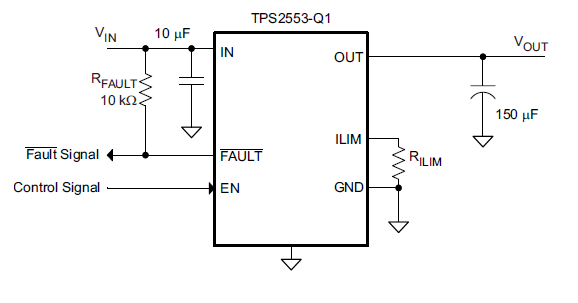If you have a related question, please click the "Ask a related question" button in the top right corner. The newly created question will be automatically linked to this question.

# TPS2553-1: The Vout in much less than the expected value

Part Number: TPS2553-1

We are implementing TPS2553-1 power switch as current limiter below a maximum current limit as shown in the figure 26 in the data sheet.The circuit parameters are as follows :

Cin = 0.1microF

Rfault = 100kOhm

Rlim = 22kOhm

Vin = 5V

The output across load is about 2.25V which is much less than the input.The voltage across the input and output pins of the TPS2553-1 is 2.75V.The output voltage decreases with the load resistance.• Hi,

With Rlim=22kohm, the current limit is about 1.2A.

The output should be about 5V.

Please measure the pin voltage of IN and OUT and also Fault pin.

It would be better also to test LIM pin voltage.

For TI's policy, I will first click resolved button.

If you have any other concern, contact me any time.

Thanks.

• The voltages for pins are as follows :

V (IN PIN) = 5V

V (OUT PIN) = 2.25 V

V (FAULT PIN) = 4.60V

V (LIM) = 2.92V

As we short the OUT pin and the ground the FAULT voltage becomes 0.48 V,the circuit also working correctly in auto retry functionality but in each case output voltage is 2.25 V when the input voltage is 5V. What is the problem ?

• Hi,

The VLIM is abnormal.